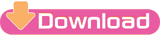POKHARA UNIVERSITY (PU)
Level: Bachelor
Program: BE
Course: Engineering Mathematics I
Semester: Spring
Year: 2017
Full Marks: 100
Pass Marks: 45
Time: 3 hrs
| DOWNLOAD PDF | Bachelor Level | BE - Engineering Mathematics I | Question Paper | 2017 | Spring Semester | Pokhara University (PU)Candidates are required to give their answers in their own words as far as practicable.
The figures in the margin indicate full marks.

Attempt ALL the questions.

1.
a) Examine continuity and differentiability at x=2 of the function
f(x) = -2+3x-x²     when 0≤x ≤2
= 2-x              when 2<x <4
Or,
State Leibniz theorem. If y=a cos(logx) + b sin(logx), show that x²yn+2 +(2n+1) xyn+1 (n²+1) yn=0. 

b) State and prove Lagrange's mean value theorem. Verify it for f(x)=x²+3x+2 at [0,2]. 

2.
a) State L'Hospital theorem and evaluate the limit: 
See More:-